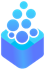# OpenDreamKit

## A project funded by the Horizon 2020

European Research Infrastructures Work Program

# 3d interactive data visualisation with K3D-jupyterK3D-jupyter is a Jupyter notebook 3d visualization package created within ODK project.

The primary aim of K3D is to be an easy tool for 3d visualization. We designed it with native interoperation with existing powerful libraries such as VTK however, it is not strictly depending on them. K3D plots are ipywidgets, thus it assures a perfect interaction of a browser side widget with Python kernel by a proven and standardized protocol.

Let us start with a simple example. The following Python code will make a surface plot of a function of two variables:

``````import k3d
import numpy as np

Nx, Ny  = 34, 33
xmin, xmax = -3, 4
ymin, ymax = -0, 3

x = np.linspace(xmin, xmax, Nx)
y = np.linspace(ymin, ymax, Ny)
x, y = np.meshgrid(x, y)
f = np.sin(x**2 + y**2)

plot = k3d.plot()
plt_surface = k3d.surface(f.astype(np.float32), bounds=[xmin,xmax,ymin,ymax])
plot += plt_surface
plot.display()
``````

The `plot` variable will be bound to an instance of the plot widget. It contains a single plot object - `plt_surface`. In the package there are many such objects available, covering most of the typical needs for 3d data visualization. They can be added to the plot in a similar way and they will appear on the same scene.

The sampled values of our function are available as an attibute of `plt_surface.heights`. Thus we can write. for example:

``````plt_surface.heights.shape
``````

Since, `plt_surface` is an ipywidget, its state on a javascript side and python are connected. We can simply assign it to other values (numpy array) and the plot will be automatically updated:

``````plt_surface.heights = np.log((x**2 + y**2))
``````

In fact, such updates are efficient enough to allow for a high degree of interactivity. One can produce animations:

``````plot.camera_auto_fit = False
for i in range(50):
plt_surface.heights = np.sin(x**2 + y**2+1.6/10*i)
``````

Such an approach can be desirable when one needs to combine server-side data processing with 3d interactive visualization. For example, in K3D we do not support unstructured volumetric meshes. There exist, however, a very powerful library for processing such data - VTK. An arbitrary unstructured mesh can be displayed at its surface which consists of triangles. The latter can be easily visualized with K3D. Therefore one can process data on server-side using VTK and send its triangularized surface representation to the plot. Since the surface data is in many cases much smaller than the volume, it allows for building responsive web applications.

The effect of such data processing are following:

The web browser gives access to the graphics hardware and allows to perform very computationally intensive visualizations. One such technique is a volume rendering. It has been implemented in K3D widget:

The flexibility of network architecture allows to easily build an HPC data visualization pipelines using jupyter notebook and webGL frontend. One can preprocess volume of data on the server and send small dataset to K3D widget for immediate inspection.

• https://github.com/K3D-tools/K3D-jupyter

<